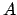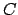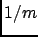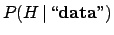Next: . Up: Constraining the mass of Previous: Outside the sensitivity region   Contents

## Including other experiments

Each of the other experiments are treated in exactly the same way. Comparingandit is interesting to see how the beam energy and the sensitivity factor contribute to constraining the mass. For reasons of space the plots are not shown. This is the rest of the Mathematica code to conclude the analysis:
(********************************************************)
(* Experiment B has been run at beam energy 0.09, with
sensitivity factor k=100,  threshold function beta^3, and
has observed 0 events.*)

kb=100
ebb=0.09
v=Sqrt[1-(m/ebb)^2]
lambda= kb*v^3
lik=Exp[-lambda]
norm=NIntegrate[lik, {m, 0, ebb}]
fb=lik/norm
avb = NIntegrate[m*fb, {m, 0, ebb}]
Plot[fb, {m, 0.07, ebb}, PlotRange->{0, 600},
AxesLabel -> {m, f}]

fbmax=fb/.m->ebb
f2b=If[m<ebb, fb, fbmax]
Plot[f2b, {m,0.07,0.15},  PlotRange->{0, 600},
AxesLabel -> {m, f}]

(* The conclusions from A + B are, with and without the condition m<ebeam,
respectively (remember that the latter is improper): *)

fcom1ab=fa*fb/NIntegrate[fa*fb, {m, 0, eba}]
avab = NIntegrate[m*fcom1ab, {m, 0, eba}]
Plot[fcom1ab, {m, 0.07, eba},  PlotRange->{0, 600},
AxesLabel -> {m, f}]
fcom2ab=f2a*f2b

(* Experiment C has been run at beam energy 0.1, with sensitivity factor k=10,
threshold function beta^3 and, has observed 0 events. *)

kc=10
ebc=0.1
v=Sqrt[1-(m/ebc)^2]
lambda= kc*v^3
lik=Exp[-lambda]
norm=NIntegrate[lik, {m, 0, ebc}]
fc=lik/norm
Plot[fc, {m, 0.07, ebc}, PlotRange->{0, 100},
AxesLabel -> {m, f}]
avc = NIntegrate[m*fc, {m, 0, ebc}]
fcmax=fc/.m->(ebc-0.000001)
f2c=If[m<ebc, fc, fcmax]
Plot[f2c, {m,0.07,0.15},  PlotRange->{0, 100},
AxesLabel -> {m, f}]
(********************************************************)The combination of the result is done in the usual way, multiplying the likelihoods or the final p.d.f.'s, if these were obtained from a uniform distribution. We only see the combination of the three experiments, shown in Fig.. Finally, the indirect determinations are also included.


(********************************************************)
(* Conclusions from A + B + C , with and without the condition m<ebeam,
respectively (remember that the latter is improper): *)

fcom1abc=f2a*f2b*fc/NIntegrate[f2a*f2b*fc, {m, 0, ebc}]
avabc=NIntegrate[m*fcom1abc, {m, 0, ebc}]
Plot[fcom1abc, {m, 0.07, ebc},  PlotRange->{0, 150},
AxesLabel -> {m, f}]
fcom2abc=f2a*f2b*f2c

(* Now we add independent determinations of m,
deriving from normal likelihoods,
and assuming uniform prior *)

g1=1/sigma1/(Sqrt[2*Pi])*Exp[-(m-mu1)^2/2/sigma1^2]
g2=1/sigma2/(Sqrt[2*Pi])*Exp[-(m-mu2)^2/2/sigma2^2]

mu1=0.09
sigma1=0.04
mu2=0.15
sigma2=0.04

(* The two overall (improper) priors may be a uniform,
or 1/m, i.e.  flat in ln(m), to express initial
uncertainty on the order of magnitude of m *)

p1=1
p2=1/m

final1=fcom2abc*g1*g2*p1/NIntegrate[fcom2abc*g1*g2*p1, {m, 0, 10}]
mean1=NIntegrate[m*final1, {m, 0, 10}]
std1=Sqrt[NIntegrate[m^2*final1, {m, 0, 10}]-mean1^2]
Plot[final1, {m, 0.0, 0.25},  PlotRange->{0, 20},
AxesLabel -> {m, f}]

final2=fcom2abc*g1*g2*p2/NIntegrate[fcom2abc*g1*g2*p2, {m, 0, 10}]
mean2=NIntegrate[m*final2, {m, 0, 10}]
std2=Sqrt[NIntegrate[m^2*final2, {m, 0, 10}]-mean2^2]
Plot[final2, {m, 0.0, 0.25},  PlotRange->{0, 20},
AxesLabel -> {m, f}]
(********************************************************)

Finally, the two extra pieces of information enable us to constrain the mass also on the upper side and to arrive at a proper distribution (see Fig.), under the condition thatexists.

From the final distribution we can evaluate, as usual, all the quantities that we find interesting to summarize the result with a couple of numbers. For a more realistic analysis of this problem see Ref. .Next: . Up: Constraining the mass of Previous: Outside the sensitivity region   Contents
Giulio D'Agostini 2003-05-15